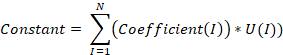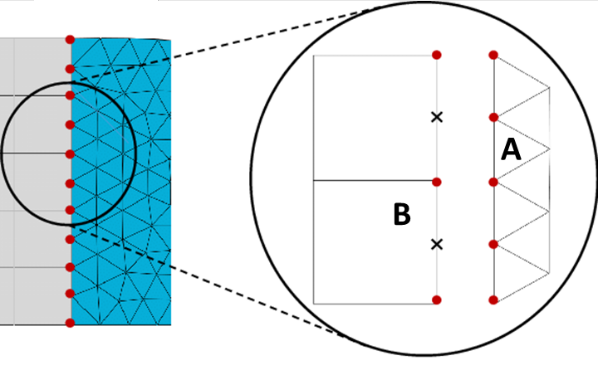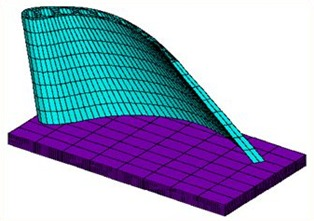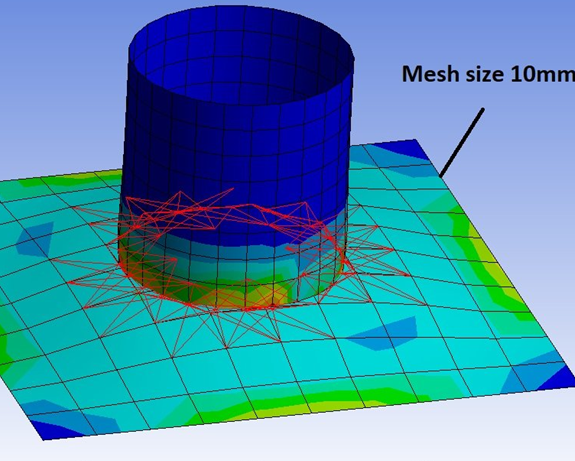# Constraint Equations# Constraint Equations

Constraint equations allow you to relate the motion of different portions of a model through the use of an equation. The equation relates the degrees of freedom (DOF) of one or more remote points for Static and Transient Structural, Harmonic and Modal analysis systems. Constraint equations are linear combinations.

Constraint relations among nodal degrees arise in finite element analysis in the applications of

1. coupling a rigid floor to its boundary nodes in a framed structure,

2. typing different types of elements together while confirming the requirement of overall compatibility

Usually, the constraint relations are implemented by performing a compatible transformation on the global matrix using the constraint equation matrix or augmenting the global equilibrium system with the constraint equations. Both approaches are far from ideal.

The former suffers from the necessity to modify the matrix profile already established, while the latter renders the global matrix ill-conditioned, unsymmetric and involves more unknowns.

To provide an elegant and practical means in implementing the constraint relation, the following factors must be considered.

1. First, the procedure must not give rise to any need for numerical treatment during equation solution or eigenanalysis, i.e., the global stiffness matrix should be well-conditioned as long as the physical problem dictates.

2. Second, the procedure must facilitate straightforward global matrix management, namely, the global matrix should not be subject to further modifications once it is formed.

This is mainly for the sake of program efficiency considering the extremely large size of the global matrix and complexity of blocking. Finally, the procedure must be easily adaptable for the current general-purpose finite element system.

The implementation of constraint relation involves consideration of 2 aspects,  namely the design of data structure and the development of modules with proper functionalities.

1. For each slave degree, only the active master degrees and their corresponding constraint coefficients need to be stored. Since these slave degrees are usually constrained by different numbers of DOFs, they will get stored in an array. Each slave degree is indicated by a negative degree-1D that points to an entry in the pointer array. The data array would contain the master degrees and their corresponding coefficients and is always accessed using the pointer array data.

2. Based on the data structure just described, modules with the following functionalities would be required that the original module for building nodal/element displacement vectors may be used without modifications.

The above statements in simple words can be described as,

CONSTRAINT EQUATIONS: allow a more complex relationship than coupled DOF.  A linear equation relating the displacements at the nodes can be created.

What is a constraint Equation?

A constraint equation (CE) defines a linear relationship between nodal degrees of freedom.

Structural : F = KU

• Every  node has a force F in each direction and a deflection

• You are solving for F & u

• The deflections are called the Degrees of freedom (u)

Constraint equations are equations that tie the value of one DOF to the value of One or more DOF’s

Added into a set of linear equations before solving

We will call them CEs most of the time

• For example, below is the connection equation for it :

• If you couple two DOFs, their relationship is simply UX1 = UX2.

• CE is a more general form of coupling and allows you to write an equation such as

UX1 + 3.5*UX2 = 10.0.

• You can define any number of CEs in a model.

• Also, a CE can have any number of nodes and any combination of DOFs.

•  Its general form is:

Coef1 * DOF+ Coef2 * DOF+ Coef3 * DOF+ ...  =  Constant

Equations fed to the solver that describe relations between DOF’s

You write equations that linearly relate one or more DOF:

Constant= I=1NCoefficientI*U(I))Where N = number of terms in the equation

U(I) = the DOF solution for the term I

After a solve, the sum of the user-defined coefficients time their deflection equals some constant.

Some background

Each CE in a model has a unique number

You define the number when you create

You refer to the CE number when you want to look at it, modify it, delete it, add to it

Most Hand CEs are pretty simple

Often an anti-symmetry

Connecting a remote mass point to your model

Connecting rotation DOFs to axial based on distances

There are 3 Types

1. Connecting adjacent regions of 2 different components with linearity - CE Interface

2. To make the part as a single unit or to consider as rigid i.e., for modeling of vehicle rim, wagon wheel, and so on – CE rigid region

3. Torque, force & moment application in 3D cylindrical models or similar pattern of models – CE load

1. CE INTERFACE:

The interface is used to "tie" together two regions with dissimilar mesh patterns by generating constraint equations that connect the selected nodes of one region to the selected elements of the other region. The command for the CE interface is CEINTF.

The process followed for the interface of 2 different components is as follows:

At the interface between regions, nodes should be selected from the denser mesh region, A, and the elements selected from the less dense mesh region, B. The degrees of freedom of region A nodes are interpolated with the corresponding degrees of freedom of the nodes on the region B elements, using the shape functions of the region B elements. Constraint equations are then written that relate region A and B nodes at the interface.Solid elements with six degrees of freedom should only be interfaced with other six-degree-of-freedom elements. The region A nodes should be near the region B elements. A location tolerance based on the smallest region B element length may be input. Stresses across the interface are not necessarily continuous. Nodes in the interface region should not have specified constraints.

Particle application of CE Interface:

1. Connecting dissimilar meshes

If two meshed objects meet at a surface but their node patterns are not the same, you can create CEs to connect them. Automatically calculates all necessary coefficients and constants

For solid elements to solid elements/ shell to shell elements, 2D or 3D1. Connecting dissimilar element types

If you need to connect element types with different DOF sets, you may need to write CEs to transfer loads from one to the other:

1. beams to solids or beams perpendicular to shells

2. shells to solids, etc.